Quadrilaterals are shapes with four sides.

In the following diagrams the arrows indicate parallel lines; the marks on lines indicate lines that are bisected; angles with the same value have the same colour; filled angles are bisected by the diagonals.

 Rectangle All four interior angles are right angles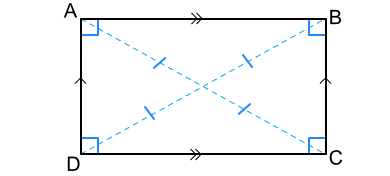Square A rectangle where all sides are of equal length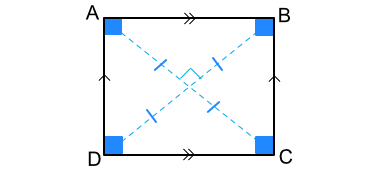Parallelogram Two sets of parallel sides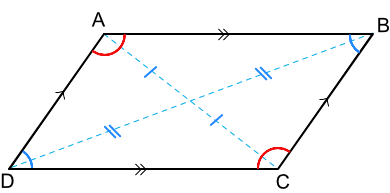Rhombus A parallelogram with equal length sides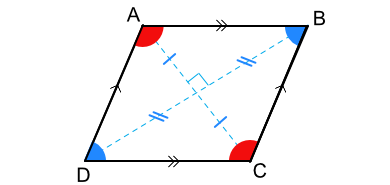Kite A quadrilateral reflected in the line BD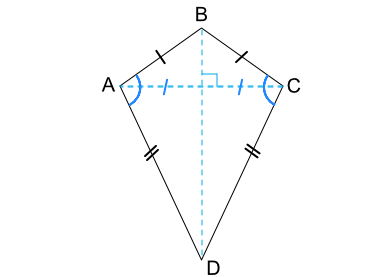Trapezium One set of parallel sidesDAB + CDA = 180ºABC + BCD = 180º## Example 1

Give one difference between a trapezium and a parallelogram.

Or the opposite angles on a parallelogram are equal, which is not true for a trapezium.

Answer: A parallelogram has two sets of parallel sides: a trapezium only has one set

## Example 2

What is the size of the angle CDA in this kite?The angle BCD, directly opposite DAB, is equal to that angle and is 100º.

There are 360º in a quadrilateral: 360 - 100 - 100 - 100 = 60º